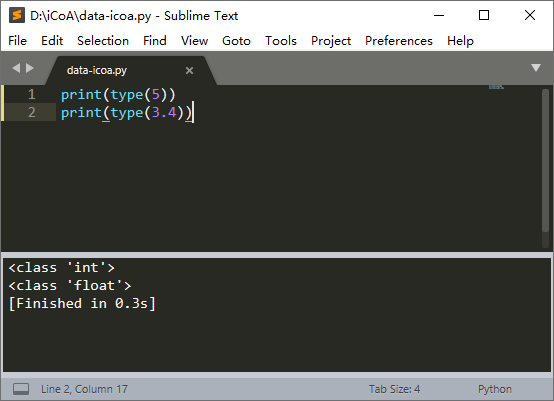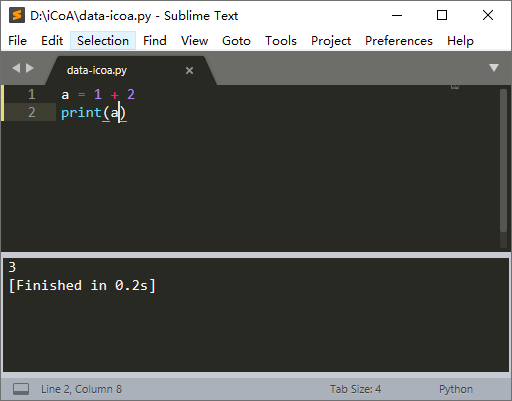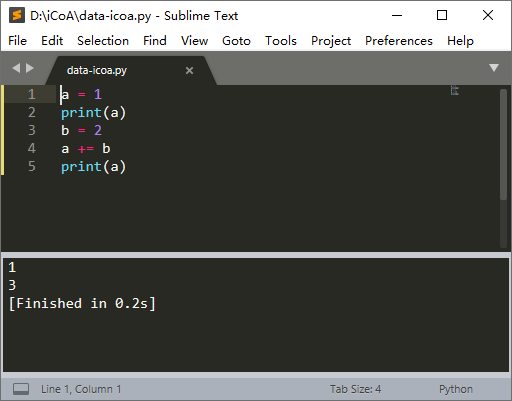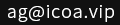您当前位置：网站首页 >> Python学习 >> Python 数字与数字型运算

# Python 数字与数字型运算

iCoA首席特工 逍遥峡谷 发布：2020-09-08 更新：2020-09-08 浏览0条评论

Python 数据类型知识中我们已经初步认识了几种 Python 中的数据类型，现在我们更详细的学习一下 数字型 以及 数字型运算。

<class 'int'>   表示是整数
<class 'float'>  表示是浮点数

```print(type(5))
print(type(3.4))```Python 中对数字进行运算用到数学运算符：

 + 加 - 减 * 乘 / 除 ** 指数 % 余数 // 整除 = 赋值

```a = 1 + 2
print(a)```

3运算符 例子 详解 += a += b 相当于 a = a + b -= a -= b 相当于 a = a - b *= a *= b 相当于 a = a * b /= a /= b 相当于 a = a / b **= a **= b 相当于 a = a ** b  指数 //= a //= b 相当于 a = a // b  整除

```a = 1
print(a)
b = 2
a += b
print(a)```

1

3```a = 2 + 1.2 + 0.8
print(a)```

4.0

 比较运算符 详解 > 前面的值 大于 后面的值 < 前面的值 小于 后面的值 >= 前面的值 大于或等于 后面的值 <= 前面的值 小于或等于 后面的值 == 前面的值 等于 后面的值 != 前面的值 不等于 后面的值

```a = 1
b = 2
c = 2
print(a == b)
print(b == c)
print(a > c)
print(b >= c)```

False

True

False

True- 相关文章 -

Python 数字与数字型运算 - 2020-09-08

Python 基础知识之数据类型 - 2020-09-07

Python 学习中非常好用的编辑器 Sublime Text 3 - 2020-09-07

Python 基础知识之变量 - 2020-09-03

Python 详细安装步骤图解 - 2020-09-01

Python 数据分析模块 Pandas 之 DataFrame 数据 - 2020-02-09

Python 数据分析模块 Pandas 之 Series 数据 - 2020-02-05

Python 数据分析第三方库 Numpy 的安装和使用 - 2020-02-03

- 文章评论 -

2874

[由于政策原因，您的留言如果提交成功后，需要审核才能展示，请改天再来查看，请不要重复提交，谢谢支持！]

- 最新评论[0条评论] -

[>>>点击查看更多评论]网络违法犯罪举报